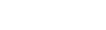﻿ TOraNumber.Compare MethodODAC

# TOraNumber.Compare MethodCompares the current TOraNumber value with the Dest value.

### Syntax

function Compare(Dest: TOraNumber): integer;

Parameters
Dest
Holds the Dest value to compare the current TOraNumber value with.
Return Value
a negative value if current number is less than Dest, zero if numbers are equal, and a positive value if the current number is greater than Dest.

### Remarks

Call the Compare method to compare the current TOraNumber value with the Dest value.

Returns a negative value if current number is less than Dest, zero if numbers are equal, and a positive value if the current number is greater than Dest.